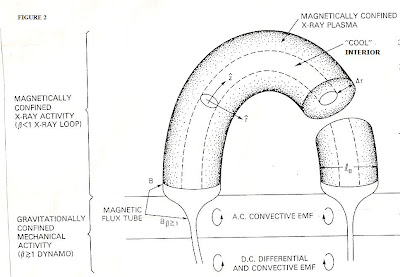## Friday, August 28, 2020

### Why The MHD Regime Is Preferred In Doing Solar PhysicsMost solar physicists work in what's called the "MHD" or magneto-hydrodynamic regime, which numerous plasma physicists have criticized as "taking a short cut". For example, treatments based on the analysis of the indigenous magnetic fields currently occupy a predominant role in analysis of energy stored in sunspot regions. The majority of these are based on MHD  theory wherein low frequency, long scale length and quasi-neutral (electric charge) assumptions are introduced into the plasma physics. Such assumptions lead to a basic set of what we call "MHD" equations, which include:

a)( r  / t)  +  Ñ (r v) = 0

b) r (  V / t)  +    r v Ñ ( v) =   -  Ñ p  + 1/c  J X B

c)  E + v X B = 0

More fundamentally, the MHD regime is really an approximation of the one-fluid and two-fluid cases, with many added simplifying assumptions – and these "take a toll"  in ignoring the kinetics and micro-physics.  In truth, MHD does represent the end product of successive information loss in the transition from Vlasov theory to two-fluid theory to one-fluid theory and thence MHD.   But the benefit of using it is self-evident in many flare models, for example, so that one is no longer hampered by being unable to "see the forest for the trees".

One starts with the Vlasov-Boltzmann equation:

f/ t + v Ñ z f + (F/ m ) Ñ v f = ( f/ t)c

Whereby the lowest moment, for example, is obtained by integrating the preceding equation with F as the Lorentz force:

ò ( f/ t)  dv    + ò  v Ñ z f dv  + (q/ m ) ò (E + v X B) f/ v  =  ò ( f/ t)c   dv

The 2nd moment is obtained by multiplying the original eqn. (Boltzmann) by mv then integrating it over dv.

The progression from using this procedure is that one gets in succession:

Two -fluid theory (e.g. ions and electrons treated as separate fluids), i.e. in fluid eqns.:

1) ( r a  / t)  +  Ñ (r a v a) = 0

where the index  a = e, i.

for electrons, ions.

2)  r a (  V o /  t)  +    r v a · Ñ ( v a) =   -  Ñ p a +  e a n a  (E + v a x B)

3)  p o  r g    =  const.

Plus the relevant form for Maxwell's equations.

More technically as Dwight R.Nicholson notes (Plasma Theory, p. 129) one takes "velocity moments of the Vlasov equation in seven dimensional (x, v, t) space."  From these (ibid.): "an infinite hierarchy of equations in 4-dimensional (x, t) space can be derived."   And: "when an appropriate truncation of this infinite hierarchy is carried out, the standard two fluid theory of plasma physics is obtained."    Thus we can proceed to treat:

f (x, v, t)  +   v · Ñ  f o  +   (q/ m) (E + v/ c  x BÑ · f o   = 0

With the normalization: o  (x, t) =  ò  df o  (x, v, t)   from which the 2 fluid velocity equations are obtained.  But given these we go direct to....
!
!
!
!
v
One fluid theory (introducing low frequency, long wave length and quasi -neutral approximations, e.g.   n e=    n i

( r  / t)  +  Ñ (r v) = 0

where no separate  index for  e, i, is needed.  Then, more quickly to...
!
!
v
MHD Theory

(This proceeds from 1-fluid theory with a few further assumptions, simplifications)

Apart from this, the most conspicuous agent of solar plasma containment and topological change is the coronal loop –Which is fully consistent with a low beta-plasma regime- since otherwise gas kinetic pressure would predominate and the configuration would be ‘open’.  Note:

beta = (½  r  v 2)/   B 2/2 m 0

where  r is the fluid density, v the fluid velocity, B the magnetic induction and m  the magnetic permeability of free space.)

With this little exercise, we see that the solar physicist is more than amply justified in employing the MHD approach.   This is directly connected to the gas kinetic pressure (½  r  v 2 being much less than the magnetic pressure  ( B 2/2 m 0 ), so the density of plasma particles  (e. ,  i ) is much less  - reducing the need to overemphasize the micro physics especially as the Vlasov collision term  ( f/  t)c  -> 0. Indeed, for most applications, i.e.  for assessing magnetic fields in  sunspot regions, two fluid theory would be far too complicated while achieving little additional insight.